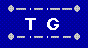## Squarematch - Cylindrical solutions 4 x 6

Cylindrical solutions have matching left and right edges, and therefore can be rotated by moving columns of tiles from one end to other, yielding another cylindrical solution.

The 4x6 solutions fall into "families", each with six members, corresponding to six rotations. One representative of each of 30 families is displayed by this applet. Since each has 6 distinct rotations, the total number is 180.

Applet Buttons:
• Next: advances to next higher number solution
• Prev: advances to next lower number solution
• <---: rotates the solution one column to the left
• --->: rotates the solution one column to the right
• Flip: rotate the solution 180°